##### TASC For Dummies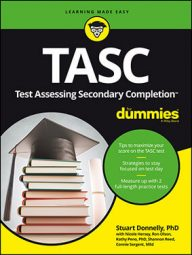Exponents are often used as a shorthand way to show repeated multiplication, so you can expect to encounter several questions on the TASC Math exam that involve exponents.

You can apply the following general rules about exponents:

• Zero exponent rule: Any number raised to the zero power is equal to 1.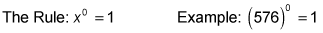• Product rule: When multiplying numbers with the same base, add the exponents.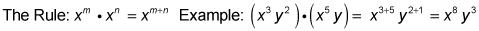• Power rule: When raising a power to a power (inside/outside exponents), multiply the exponents.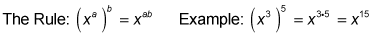• Quotient rule: When dividing numbers with the same base, subtract the exponent of the denominator from the exponent of the numerator.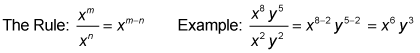• Negative exponent rule 1: A negative exponent in the numerator indicates that part of the term belongs in the denominator.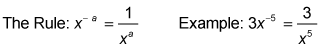• Negative exponent rule 2: A negative exponent in the denominator indicates that part of the term belongs in the numerator.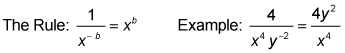• Negative exponent rule 3: When raising an entire quotient to a negative exponent, you can "flip" the fraction (use the reciprocal).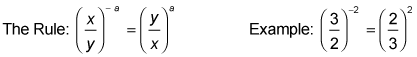• Distribution rule 1: When raising an entire product to a power, distribute the exponent to each part of the product.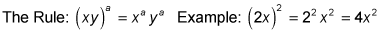• Distribution rule 2: When raising an entire quotient to a power, distribute the exponent to each part of the quotient.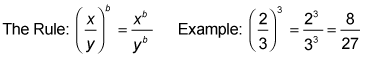Keep in mind that you should never distribute over addition or subtraction! For example,

it really means (x + y)2 = (x + y)(x + y).

## Practice questions

1. Which is equivalent to (x2)1/3?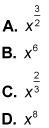2. Simplify 3(20 + 52). A. 21 B. 31 C. 75 D. 78

1. The correct answer is Choice (C). Using the power rule for exponents you multiply the inside/outside exponents. Multiplying the exponents results in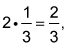which means the answer is Choice (C), x2/3.
2. The correct answer is Choice (D). You should notice that you must use both the order of operations and the exponent rules. Recall that anything to the 0 power is 1, so using the exponent rules you get 3(1 + 25) = 3(26) = 78, which is Choice (D). Choices (A) and (C) result from applying the 0 exponent rule incorrectly.

Stuart Donnelly, PhD, was awarded a PhD in mathematics from Oxford University. He has prepared students for the TASC test and GED Test for the past two decades.

Stuart Donnelly, PhD, earned his doctorate in mathe-matics from Oxford University at the age of 25. Since then, he has established successful tutoring services in both Hong Kong and the United States and is considered by leading educators to be one of the most experienced and qualified private tutors in the country.

Stuart Donnelly, PhD, was awarded a PhD in mathematics from Oxford University. He has prepared students for the TASC test and GED Test for the past two decades.

Stuart Donnelly, PhD, was awarded a PhD in mathematics from Oxford University. He has prepared students for the TASC test and GED Test for the past two decades.

Sandra Luna McCune, PhD, is professor emeritus and a former Regents professor at Stephen F. Austin State University. She's now a full-time author. Shannon Reed, MA, MFA, is a visiting lecturer at the University of Pittsburgh, where she teaches composition, creative writing, and business writing.

Stuart Donnelly, PhD, was awarded a PhD in mathematics from Oxford University. He has prepared students for the TASC test and GED Test for the past two decades.